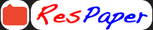Trending ▼   ResFinder# 10 diamind jubilee physics

5 pages, 60 questions, 0 questions with responses, 0 total responses,10sonalirajiv  City- Mumbai +Fave Message ProfileTimelineUploads
 Home > sonalirajiv >Formatting page ...

Diamond Jubilee High School Preliminary Examination(SET A) Std : X Science Paper I - Physics Date : 18-1-2021 Marks : 80 Time: 2 hr Answer to this Paper must be written on the paper provided separately. You will not be allowed to write during the first 10 minutes. This time is to be spent in reading the Question Paper. The time given at the head of this Paper is the time allowed for writing the answers. Attempt all questions from Section A and any four questions from Section B. The intended marks for questions or parts of questions are given in brackets [ ] This paper consists of five sides Section A (40 marks) (All questions are compulsory) Question 1  a) i) Name the physical quantity also measured in kWh. ii)Give the relation between kWh and the SI unit of the quantity mentioned in (i) above. b) Mechanical energy is the sum of two forms of energy A and B. Form A depends on the velocity of the body while form B whenever put to use can be changed only to form A. Identify the forms of Energy. c) Why is a jack screw provided with a long arm? State the formula to support your answer. d) i) What should be the angle between force and displacement to get maximum work? ii) If a body revolves in a circular path under the influence of a force, what is the work done by the force on completing 100 rounds? e) State two factors on which the lateral displacement produced by a glass slab depends? Question 2  a) If the power of a motor is 100 kW, then at what speed can it raise a load of 50000 N? b) i) If a machine is said to be 80% efficient, then where is the remaining energy lost? ii)Calculate the ideal mechanical advantage of a lever in which the effort arm is 60 cm and the load arm is 4 cm. c) Given below is the V-I Graph of a conductor. Identify which type of a conductor it is and give one example of it. Std : 10 Subject : Physics Page : 1Formatting page ...Formatting page ...Formatting page ...Formatting page ...